### Strain Measures: Uniaxial Strain Measures

#### Learning Outcomes

• Describe three different uniaxial strain measures: Engineering, true, and Green and their relationship with the stretch.
• Calculate the three uniaxial strain measures using their respective formulas.

In this section, we give various definitions and interpretations of strain measures. We will start the section with the definition of uniaxial strains:

### Uniaxial Strain Measures

#### Definitions

Assume that a bar of length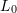is uniformly stretched to a length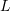. The quantity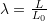is termed the stretch ratio. There are various uniaxial strain measures that we can use to define how the length of the bar changes:

The Engineering Strain is defined as: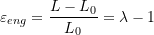The True (Logarithmic) Strain is defined as: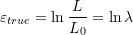The Green (Lagrangian) Strain is defined as: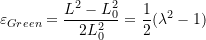The stretch ratio has the limits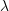, which limits the strain measures as follows: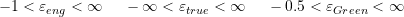The above three strain measures are related as follows:There are advantages to each of the above strain measures. The engineering strain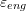is the most intuitive measure as it is simply the ratio of the change in length over the original length. The true strainis additive which led to the terminology: “True”! For example, if a bar is stretch fromto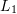and then to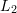. Then, the true strain measured fromtois also equal to the sum of the true strain fromtoplus the true strain fromto.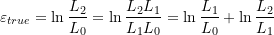The Green strain, on the other hand, can easily be computed in a three dimensional object with nonlinear deformation.

There is a very subtle difference between the engineering and the true strain measures when viewed as integrals. The true strain is the integral of the function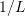while the engineering strain is the integral of the function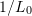: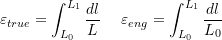The following tool draws the variation of the above three strain measures when the stretch ratio varies between 0 and 2. By changing the slider you can also pick a value for the stretch ratio between 0 and 2 and the tool will display the corresponding values of the strain measures. Notice that the three strain measures agree when the stretch ratio is close to 1, i.e., for small strains, the three measures agree.

#### Nonuniform Axial Strain

In the above discussion, we assumed that the bar deforms uniformly. I.e., the extension or contraction is the same at every point on the bar. However, a more general case is when the bar deforms non-uniformly. In this case, we can assume that the original position of the bar is given by a function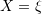wherevaries between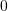and. The new position is given by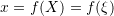. We can think of the local stretch as a the limit: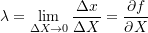In this case, the three strain measures would be given by: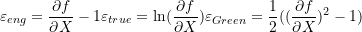##### EXAMPLE:

Assume a bar of length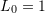with the ends situated at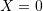and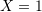. Assuming that the new position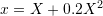, then, the stretch is different at every point and is given by: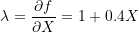I.e., the stretch is zero at the end, while is the highest at the end. In this case, the strain is not uniformly distributed, but rather is given by the functions: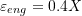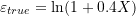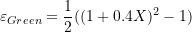The following tool draws the sketches of the reference and deformed configurations. The vertical lines in the reference configuration are equidistant but they are not after deformation. Change the density of the vertical lines and notice that after deformation, the distances between the vertical lines on the right side of the bar are more than the distances on the left side.

The following tool draws the distribution of the strain as a function of the original position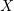. As predicted, the strain on the left side of the bar after deformation, is higher than the strain on the right side. You can change the slider to pick a specific value forfor which the tool will calculate and display the value of the different measures of strain.